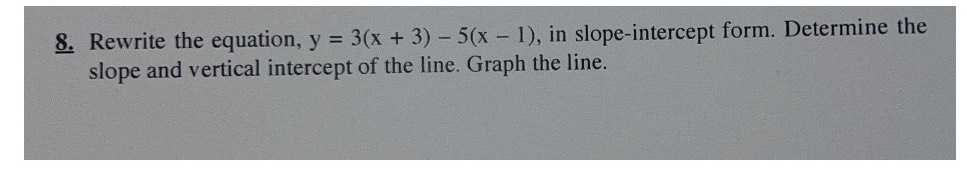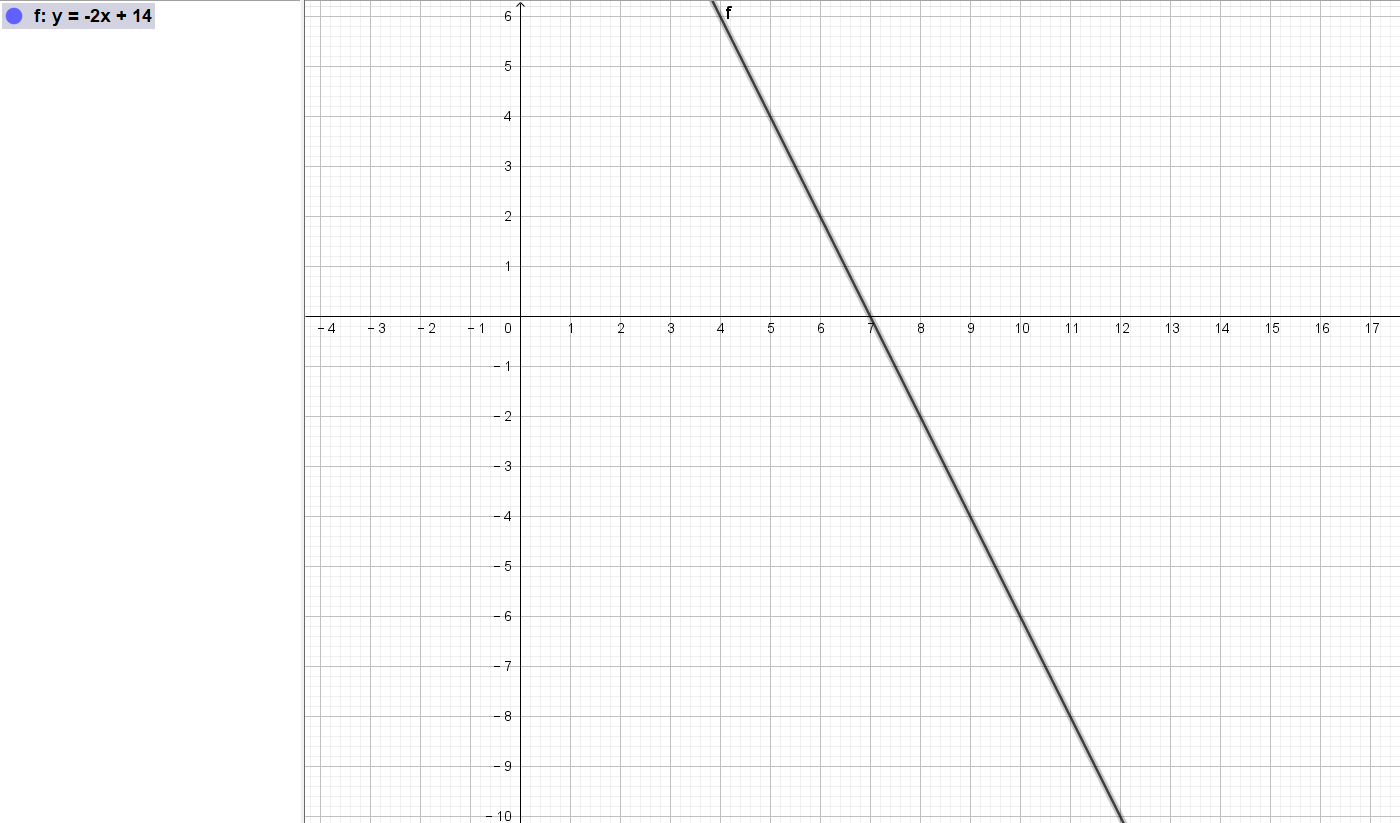### ¿Todavía tienes preguntas de matemáticas?

Pregunte a nuestros tutores expertos
Algebra
Pregunta8. Rewrite the equation, y = 3(x + 3) - 5(x - 1), in slope-intercept form. Determine the slope and vertical intercept of the line. Graph the line. graph the line and rewrite the equation in slope-intercept form. show your work please :)

$$y= -2x+ 14$$,the slope is $$- 2$$ and the intercept is $$14$$.